# NCERT Solutions for Class 10 Maths Exercise 1.2## myCBSEguide App

CBSE, NCERT, JEE Main, NEET-UG, NDA, Exam Papers, Question Bank, NCERT Solutions, Exemplars, Revision Notes, Free Videos, MCQ Tests & more.

NCERT solutions for Class 10 Maths Real Numbers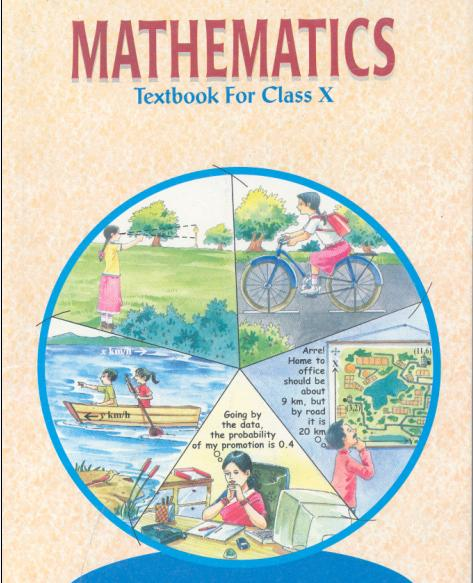## NCERT Solutions for Class 10 Maths Real Numbers

###### 1. Express each number as product of its prime factors:

(i) 140

(ii) 156

(iii) 3825

(iv) 5005

(v) 7429

Ans. (i) 140 =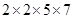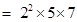(ii) 156 =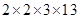=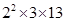(iii) 3825 =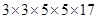=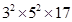(iv) 5005 =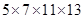(v) 7429 =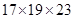###### 2. Find the LCM and HCF of the following pairs of integers and verify that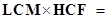product of the two numbers.

(i) 26 and 91

(ii) 510 and 92

(iii) 336 and 54

Ans. (i) 26 and 91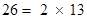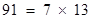HCF = 13

LCM =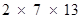= 182

Product of two numbers 26 and 91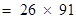= 2366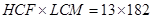= 2366

Hence, product of two numbers =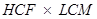(ii) 510 and 92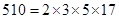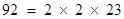HCF = 2

LCM =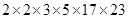= 23460

Product of two numbers 510 and 92 =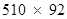= 46920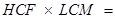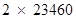= 46920

Hence, product of two numbers =(iii) 336 and 54

336=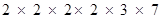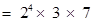54 =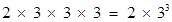HCF =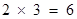LCM =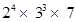= 3024

Product of two numbers 336 and 54 =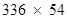= 18144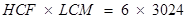= 18144

Hence, product of two numbers =###### 3. Find the LCM and HCF of the following integers by applying the prime factorisation method.

(i) 12, 15 and 21

(ii) 17, 23 and 29

(iii) 8, 9 and 25

Ans. (i) 12, 15 and 21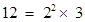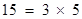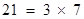HCF = 3

LCM =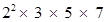= 420

(ii) 17, 23 and 29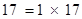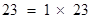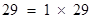HCF = 1

LCM =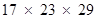= 11339

(iii) 8, 9 and 25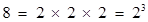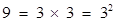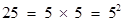HCF = 1

LCM =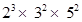= 1800

NCERT Solutions for Class 10 Maths Exercise 1.2

###### 4. Given that HCF (306, 657) = 9, find LCM (306, 657).

Ans. HCF (306, 657) = 9

We know that, LCM × HCF = Product of two numbers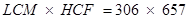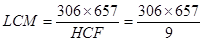LCM = 22338

NCERT Solutions for Class 10 Maths Exercise 1.2

###### 5. Check whether 6n can end with the digit 0 for any natural number n.

Ans. If any number ends with the digit 0, it should be divisible by 10.

In other words, it will also be divisible by 2 and 5 as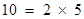Prime factorisation of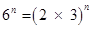It can be observed that 5 is not in the prime factorisation of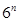.

Hence, for any value of n,will not be divisible by 5.

Therefore,cannot end with the digit 0 for any natural number n.

NCERT Solutions for Class 10 Maths Exercise 1.2

###### 6. Explain why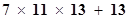and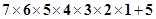are composite numbers.

Ans. Numbers are of two types – prime and composite.

Prime numbers can be divided by 1 and only itself, whereas composite numbers have factors other than 1 and itself.

It can be observed that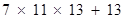=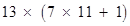=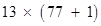=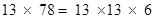The given expression has 6 and 13 as its factors.

Therefore, it is a composite number.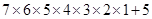=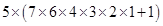=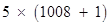=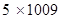1009 cannot be factorized further

Therefore, the given expression has 5 and 1009 as its factors.

Hence, it is a composite number.

NCERT Solutions for Class 10 Maths Exercise 1.2

###### 7. There is a circular path around a sports field. Sonia takes 18 minutes to drive one round of the field, while Ravi takes 12 minutes for the same. Suppose they both start at the same point and at the same time, and go in the same direction. After how many minutes will they meet again at the starting point?

Ans. It can be observed that Ravi takes lesser time than Sonia for completing 1 round of the circular path. As they are going in the same direction, they will meet again at the same time when Ravi will have completed 1 round of that circular path with respect to Sonia. And the total time taken for completing this 1 round of circular path will be the LCM of time taken by Sonia and Ravi for completing 1 round of circular path respectively i.e., LCM of 18 minutes and 12 minutes.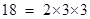And,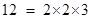LCM of 12 and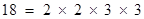= 36

Therefore, Ravi and Sonia will meet together at the starting point after 36 minutes.

## NCERT Solutions for Class 10 Maths Exercise 1.2

NCERT Solutions Class 10 Maths PDF (Download) Free from myCBSEguide app and myCBSEguide website. Ncert solution class 10 Maths includes text book solutions from Mathematics Book. NCERT Solutions for CBSE Class 10 Maths have total 15 chapters. 10 Maths NCERT Solutions in PDF for free Download on our website. Ncert Maths class 10 solutions PDF and Maths ncert class 10 PDF solutions with latest modifications and as per the latest CBSE syllabus are only available in myCBSEguide.

## CBSE app for Class 10

To download NCERT Solutions for Class 10 Maths, Computer Science, Home Science,Hindi ,English, Social Science do check myCBSEguide app or website. myCBSEguide provides sample papers with solution, test papers for chapter-wise practice, NCERT solutions, NCERT Exemplar solutions, quick revision notes for ready reference, CBSE guess papers and CBSE important question papers. Sample Paper all are made available through the best app for CBSE students and myCBSEguide website.### 17 thoughts on “NCERT Solutions for Class 10 Maths Exercise 1.2”

1. Thanks

2. Thanks

3. Nice god and meaningful solutions and on other sites there is not proper solution

4. Thankyou guide

5. Brilliant

6. Thnx

7. Nice

8. NICE

9. Good

10. Thanksssss